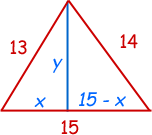SEARCH HOMEMath Central Quandaries & QueriesQuestion from William, a student: I need to find the height of a triangle if the base is 15, one side is 14 and the other side is 13.we have two responses for you

William,

I have two methods to suggest. One is to use Pythagoras' Theorem and some algebra. This method is illustrated in the answer I gave to Sharon in response to a question she asked last year. The second method uses Heron's formula. Heron's formula shows you how to find the area of a triangle from the lengths of the three sides. Use Heron's formula to find the area A of your triangle. You also know that the area is half the base times the height and since you have a base of 15

A = 1/2 ×15 × height.

Solve for the height.

I hope this helps,
Penny

Hi WilliamHi William.

If you use the Pythagorean Theorem for each right triangle and solve each for y2, you can come up with an equation with just x in it and solve for x either by factoring or by using the Quadratic Formula. Then you can use that value of x to find y.

Cheers,
Stephen La Rocque.Math Central is supported by the University of Regina and The Pacific Institute for the Mathematical Sciences.